13 C
Jaipur
Monday, January 25, 2021

# C++ Bitwise Operators – Linux Hint

### Tesla Sues Former Employee for Stealing Sensitive Data

In this article, we are going to discuss bitwise operators in the C++ programming language. We will see several working examples to understand bitwise operations in detail. In C++, the bitwise operators work on the individual bit level.

## Brief Overview of Bitwise Operators

An operator is a symbol that instructs the compiler to perform certain mathematical or logical operations. There are several types of operators in C++, such as:

1. Arithmetic Operators
2. Logical Operators
3. Relational Operators
4. Assignment Operators
5. Bitwise Operators
6. Misc Operators

All the Bitwise operators work at the individual bit level. The bitwise operator can only be applied to the integer and character data types. For example, if you have an integer type variable with the size of 32 bits and you apply bitwise NOT operation, the bitwise NOT operator will be applied for all 32 bits. So, eventually, all the 32 bits in the variable will be inversed.

There are six different bitwise operators are available in C++:

1. Bitwise OR [represented as “|”]
2. Bitwise AND [represented as “&”]
3. Bitwise NOT [represented as “~”]
4. Bitwise XOR [represented as “^”]
5. Bitwise Left Shift [represented as “<<”]
6. Bitwise Right Shift [represented as “>>”]

## Bitwise OR Truth Table

The Bitwise OR operator produces 1 when at least one operand is set to 1. Here is the truth table for the Bitwise OR operator:

Bit-1 Bit-2 Bit-1 | Bit-2
0 0 0
0 1 1
1 0 1
1 1 1

## Bitwise AND Truth Table

Bitwise AND operator produces 1 when both the operands are set to 1. Here is the truth table for the Bitwise AND operator:

Bit-1 Bit-2 Bit-1 & Bit-2
0 0 0
0 1 0
1 0 0
1 1 1

## Bitwise NOT Truth Table

Bitwise NOT operator inverts the operand. Here is the truth table for Bitwise NOT operator:

## Bitwise XOR Truth Table

Bitwise XOR operator produces 1 if, and only if, one of the operands is set to 1. Here is the truth table for Bitwise AND operator:

Bit-1 Bit-2 Bit-1 ^ Bit-2
0 0 0
0 1 1
1 0 1
1 1 0

## Bitwise Left Shift Operator

Bitwise Left Shift operator shifts all the bits left by the specified number of specified bits. If you left shift all the bits of the data by 1, the original data will be multiplied by 2. Similarly, if you left shift all the bits of the data by 2, the original data will be multiplied by 4.

## Bitwise Right Shift Operator

Bitwise Right Shift operator shifts all the bits right by the specified number of specified bits. If you right shift all the bits of the data by 1, the original data will be divided (integer division) by 2. Similarly, if you right shift all the bits of the data by 2, the original data will be divided (integer division) by 4.

## Examples

Now, since we have understood the basic concept of bitwise operations, let us look at a couple of examples, which will help you to understand the bitwise operations in C++:

• Example-1: Bitwise OR Operator
• Example-2: Bitwise AND Operator
• Example-3: Bitwise NOT Operator
• Example-4: Bitwise XOR Operator
• Example-5: Bitwise Left Shift Operator
• Example-6: Bitwise Right Shift Operator
• Example-7: Set Bit
• Example-8: Clear Bit

The example-7 and 8 are for demonstrating the real-world usage of bitwise operators in the C++ programming language.

## Example-1: Bitwise OR Operator

In this example program, we will demonstrate the Bitwise OR operator.

#include <iostream>
#include <string>
#include <bitset>
using namespace std;

// display() function
void display(string print_msg, int number)
{
bitset<16> myBitSet(number);

cout << print_msg;
cout << myBitSet.to_string() << ” (“ << myBitSet.to_ulong() << “) “ << endl;
}

int main()
{
int first_num = 7, second_num = 9, result = 0;

// Bitwise OR operation
result = first_num | second_num;

// print the input numbers
cout << endl;
display(“First Number is        =  “, first_num);
display(“Second Number is       =  “, second_num);

// print the output value
display(“first_num | second_num =  “, result);
cout << endl;

return 0;
}

## Example-2: Bitwise AND Operator

In this example program, we will illustrate Bitwise AND operator.

#include <iostream>
#include <string>
#include <bitset>
using namespace std;

// display() function
void display(string print_msg, int number)
{
bitset<16> myBitSet(number);

cout << print_msg;
cout << myBitSet.to_string() << ” (“ << myBitSet.to_ulong() << “) “ << endl;
}

int main()
{
int first_num = 7, second_num = 9, result = 0;
// Bitwise AND operation
result = first_num & second_num;

// print the input numbers
cout << endl;
display(“First Number is        =  “, first_num);
splay(“Second Number is       =  “, second_num);

// print the output value
display(“first_num & second_num =  “, result);
cout << endl;

return 0;
}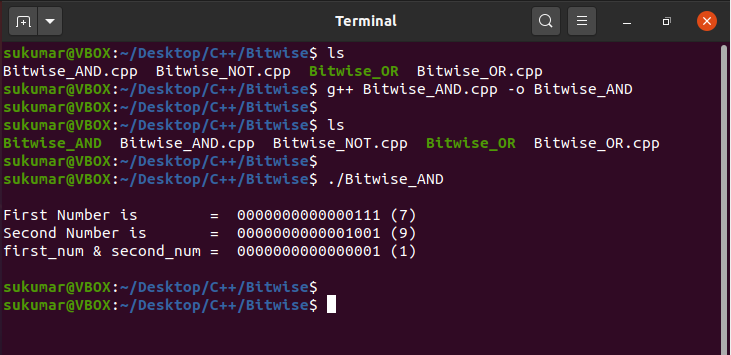## Example-3: Bitwise NOT Operator

In this example program, we will understand how Bitwise NOT operator works in C++.

#include <iostream>
#include <string>
#include <bitset>
using namespace std;

// display() function
void display(string print_msg, int number)
{
bitset<16> myBitSet(number);
cout << print_msg;
cout << myBitSet.to_string() << ” (“ << myBitSet.to_ulong() << “) “ << endl;
}

int main()
{
int first_num = 7, second_num = 9, result_1 = 0, result_2 = 0;

// Bitwise NOT operation
result_1 = ~first_num;
result_2 = ~second_num;

// print the input numbers and output value
cout << endl;
display(“First Number is    =  “, first_num);
display(“~first_num         =  “, result_1);
cout << endl;

// print the input numbers and output value
display(“Second Number is   =  “, second_num);
display(“~second_num        =  “, result_2);

cout << endl;

return 0;
}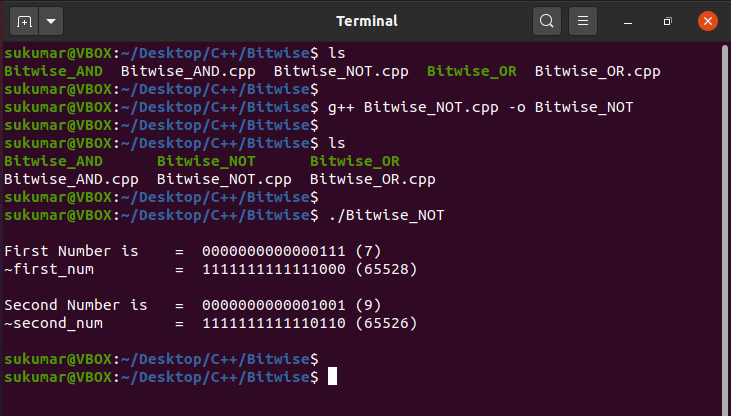## Example-4: Bitwise XOR Operator

This program intends to explain how the Bitwise XOR operator works in C++.

#include <iostream>
#include <string>
#include <bitset>
using namespace std;

// display() function
void display(string print_msg, int number)
{
bitset<16> myBitSet(number);
cout << print_msg;
cout << myBitSet.to_string() << ” (“ << myBitSet.to_ulong() << “) “ << endl;
}

int main()
{
int first_num = 7, second_num = 9, result = 0;

// Bitwise XOR operation
result = first_num ^ second_num;

// print the input numbers
cout << endl;
display(“First Number is        =  “, first_num);
display(“Second Number is       =  “, second_num);

// print the output value
display(“first_num ^ second_num =  “, result);
cout << endl;

return 0;
}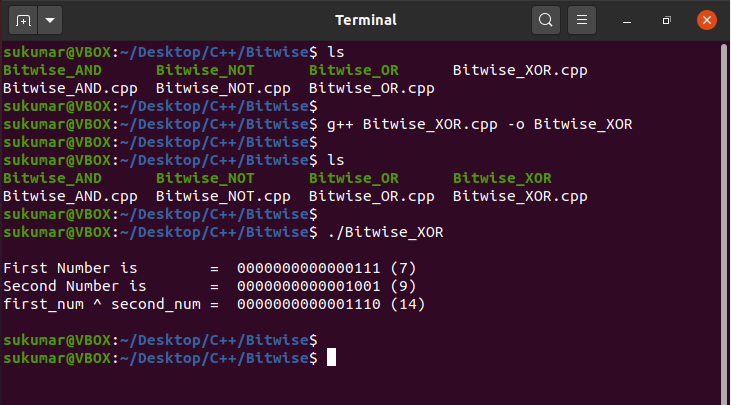## Example-5: Bitwise Left Shift Operator

Now, we will see the example of the Bitwise Left Shift operator. In this program, we have declared two numbers, first_num and second_num of integer type. Here, the “first_num” is left-shifted by one bit, and the “second_num” is left-shifted by two bits.

#include <iostream>
#include <string>
#include <bitset>
using namespace std;

// display() function
void display(string print_msg, int number)
{
bitset<16> myBitSet(number);
cout << print_msg;
cout << myBitSet.to_string() << ” (“ << myBitSet.to_ulong() << “) “ << endl;
}

int main()
{
int first_num = 7, second_num = 9, result_1 = 0, result_2 = 0;

// Bitwise Left Shift operation
result_1 = first_num << 1;
result_2 = second_num << 2;

// print the input numbers and output value
cout << endl;
display(“First Number is    =  “, first_num);
display(“first_num << 1     =  “, result_1);
cout << endl;

// print the input numbers and output value
display(“Second Number is   =  “, second_num);
display(“second_num << 2    =  “, result_2);

cout << endl;

return 0;
}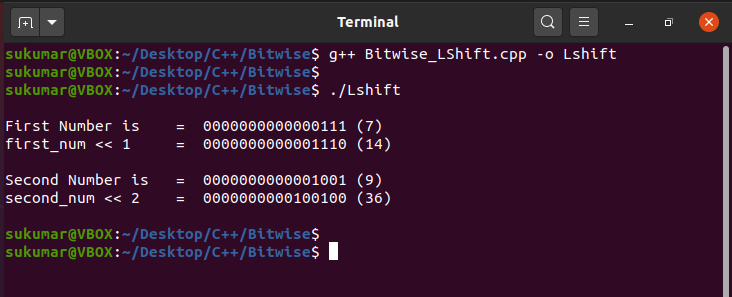## Example-6: Bitwise Right Shift Operator

Now, we will see another example to understand the Bitwise Right Shift operator. We have declared two numbers, first_num and second_num of integer type. Here, the “first_num” is right-shifted by one bit, and the “second_num” is right-shifted by two bits.

#include <iostream>
#include <string>
#include <bitset>
using namespace std;

// display() function
void display(string print_msg, int number)
{
bitset<16> myBitSet(number);
cout << print_msg;
cout << myBitSet.to_string() << ” (“ << myBitSet.to_ulong() << “) “ << endl;
}

int main()
{
int first_num = 7, second_num = 9, result_1 = 0, result_2 = 0;

// Bitwise Right Shift operation
result_1 = first_num >> 1;
result_2 = second_num >> 2;

// print the input numbers and output value
cout << endl;
display(“First Number is    =  “, first_num);
display(“first_num >> 1     =  “, result_1);
cout << endl;

// print the input numbers and output value
display(“Second Number is   =  “, second_num);
display(“second_num >> 2    =  “, result_2);

cout << endl;

return 0;
}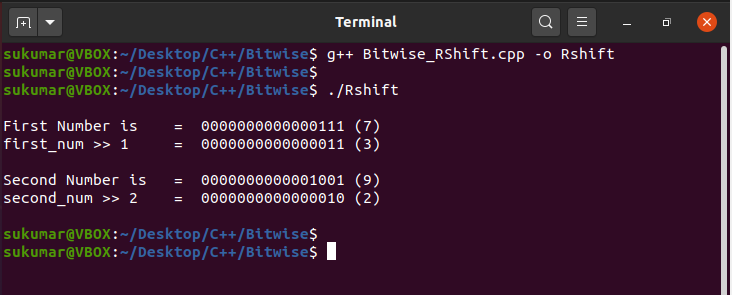## Example-7: Set Bit

This example intends  to show how to set a particular bit using bitwise operators.

#include <iostream>
#include <string>
#include <bitset>
using namespace std;

// display() function
void display(string print_msg, int number)
{
bitset<16> myBitSet(number);
cout << print_msg;
cout << myBitSet.to_string() << ” (“ << myBitSet.to_ulong() << “) “ << endl;
}

int main()
{
int first_num = 7, second_num = 9;

// print the input number – first_num
cout << endl;
display(“First Number is           =  “, first_num);

// Set 5th bit
first_num |= (1UL << 5);

// Print output
display(“Set 5th bit of first_num  =  “, first_num);
cout << endl;

// print the input number – second_num
cout << endl;
display(“Second Number is           =  “, second_num);// Set 6th bit
second_num |= (1UL << 6);

// Print output
display(“Set 6th bit of second_num  =  “, second_num);
cout << endl;

return 0;
}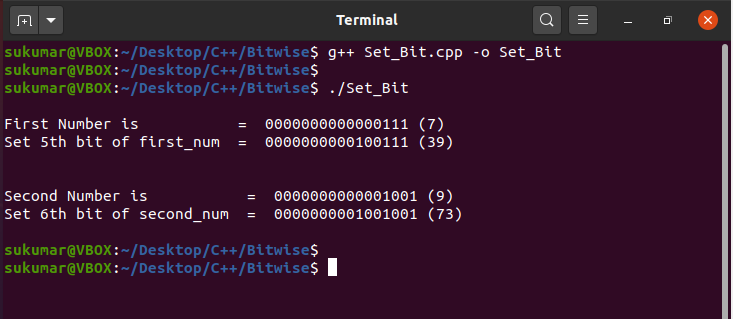## Example-8: Clear Bit

This example intends to show how to clear a particular bit using bitwise operators.

#include <iostream>
#include <string>
#include <bitset>
using namespace std;

// display() function
void display(string print_msg, int number)
{
bitset<16> myBitSet(number);
cout << print_msg;
cout << myBitSet.to_string() << ” (“ << myBitSet.to_ulong() << “) “ << endl;
}

int main()
{
int first_num = 7, second_num = 9;

// print the input number – first_num
cout << endl;
display(“First Number is           =  “, first_num);

// Clear 2nd bit
first_num &= ~(1UL << 2);

// Print output
display(“Set 2nd bit of first_num  =  “, first_num);
cout << endl;

// print the input number – second_num
cout << endl;
display(“Second Number is           =  “, second_num);

// Clear 3rd bit
second_num &= ~(1UL << 3);

// Print output
display(“Set 3rd bit of second_num  =  “, second_num);
cout << endl;

return 0;
}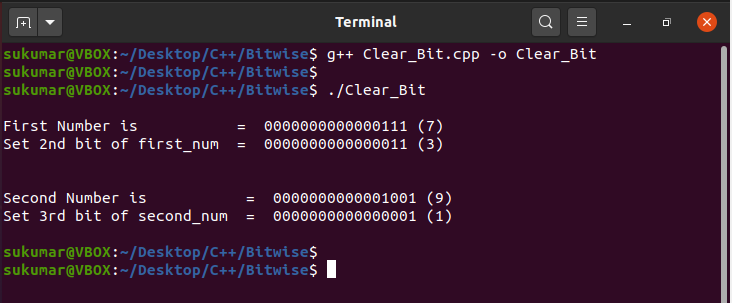## Conclusion

The bitwise operator is primarily used to manipulate the individual bits for integer and character data type. The bitwise operator is heavily used in embedded software development. So, if you are developing a device driver or a system very close to the hardware level, you may want to use these bitwise operators.